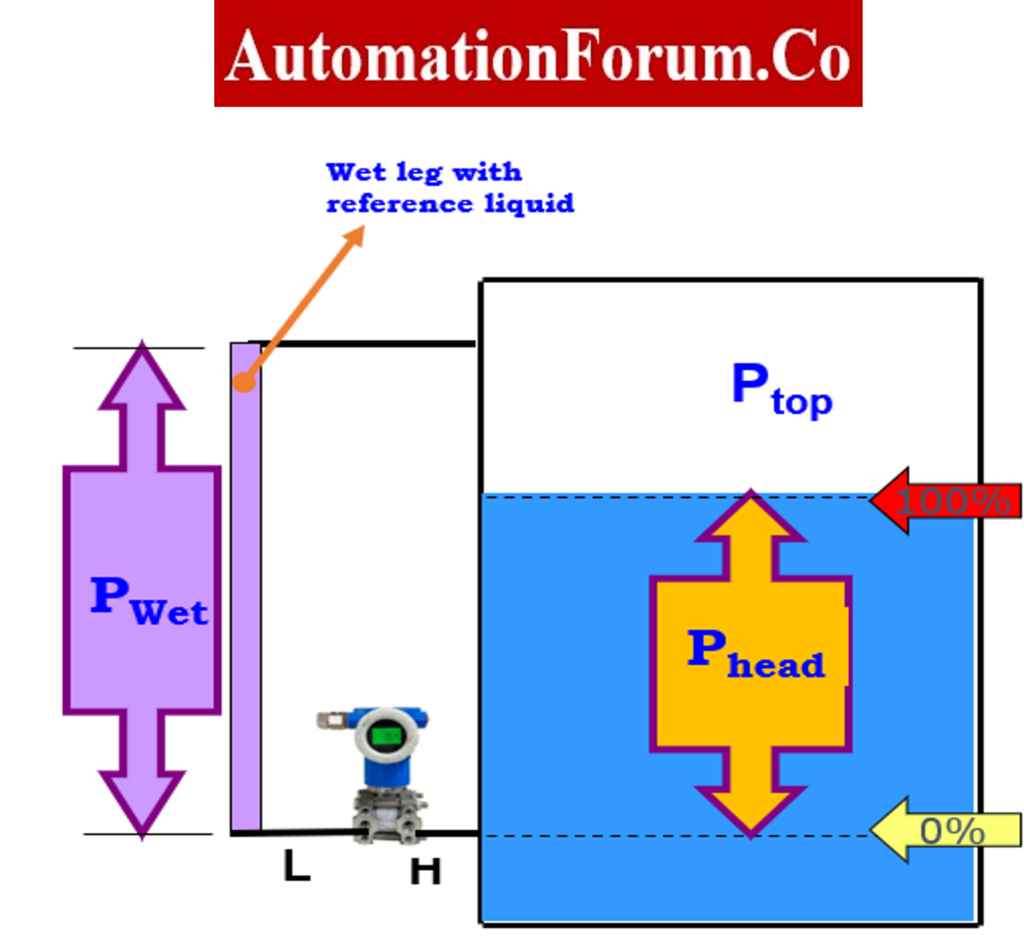# Zero Suppression and Zero Elevation## What is Hydrostatic Pressure?

### Theory of Hydrostatic Pressure:

If we consider a little cube of liquid that is at rest below a free surface, then the pressure that is created by the height of the liquid that is above this small cube must be balanced by a pressure that is resisting being applied to it.

Because of this, the stress is the same in all directions for an extremely tiny cube, and the liquid weight or equivalent pressure may be represented as

## What is the Hydrostatic Pressure Formula?

P = ρ * g * h

P = Hydrostatic Pressure (Pa)

ρ = Density (kg/m3)

g = Gravity (m/s2)

H = Height (mm)

P  =  SG * h

P = Hydrostatic Pressure (mmH2O)

SG = Specific gravity

H = Height (mm)

## Level Measurement by Hydrostatic Pressure

Differential pressure (DP) is often used for liquids that are clean and have the same specific gravity. The differential pressure transmitters are used for measuring level in a vessel by hydrostatic pressure. DP transmitters don’t measure level directly. Instead, they figure out the level by measuring the pressure of the liquid pushing down on a diaphragm. This is called hydrostatic pressure. Changes in the temperature or specific gravity of the medium will cause big mistakes. If the vessel is under pressure, you need to add a second connection above the liquid to measure and correct this variable. This is why the difference between these two points is called differential pressure.

## How do you Measure Tank Level with a DP Transmitter?

Theory of Open Tank Level Measurement:  The differential pressure transmitter will measure the pressure corresponding to the height of the fluid above it when it is installed near the bottom of the tank by the high side, and it will measure the pressure at atmospheric level  by the low side. The LP side of the transmitter is kept open to atmospheric pressure.

## How to Measure the Level using a Differential Pressure Transmitter in an Open Tank?

For example the DP transmitter installed in a zero-based direct system, the calibration range may be computed using the following method. In open Vessel Level Measurement (Direct mount), When has liquid at 0% we get

Phigh  = 0 + Patm

Plow   = Patm

ΔP at 0%  = Phigh – Plow

= 0 + Patm – Patm

= 0

When has liquid at 100% we get

Plow   = Patm

ΔP at 100%= Phigh – Plow

= Phead + Patm – Patm

Calibration Range = ΔP at 0% to ΔP at 100%

4 mA point  =  [ΔP at 0% ]

20 mA point  =  [ΔP at 100% ]

## What is “Zero Suppression” in Instrumentation?

Theory of Zero Suppression: When the transmitter is mounted below the vessel connection, “zero suppression” is often used to reduce the range of the liquid head in the pipe that connects the transmitter to the tank. A suppressed zero range is one in which the value of the measured variable zero is less than the value of the lower range.

## What is Zero Elevation in terms of Level Measurement using Pressure?

Theory of Zero Elevation: “Zero Elevation” is needed for transmitters that are mounted above the high side tap. In this case, the transmitter reads a negative pressure when the tank is empty because of the head effect of the capillary fill fluid. In an elevated zero range, the value of the measure variable zero is higher than the value of the lower range..

Note that this configuration is not recommended because the DP transmitter tends to be unstable when the liquid level gets below the DP transmitter level, which can cause bubble traps if a seal system is not used.

## What is the Dry Leg Level Measurement Method?

### Theory of  Closed Tank Level Measurement

Closed Tank Level Measurement with Dry Leg:  There is no fluid in the low-side impulse piping or leg. If the gas above the fluid doesn’t condense, the pipes on the low side of the transmitter will stay empty. Sometimes a heat trace system is applied to the pipe on the dry leg.

For example the transmitter is installed in  Zero-Based Remote Mount, The Calibration Range may be computed using the following method

Phigh  = 0 + Ptop

Plow   = Ptop

ΔP at 0%  = Phigh – Plow

= 0 + Ptop – Ptop

= 0

The pressure on the HP side of the transmitter is equal to the sum of process pressure (Ptop) and liquid head pressure (Phead).

When has liquid at 100% we get

Plow   = Ptop

ΔP at 100%= Phigh – Plow

= Phead + Ptop – Ptop

Calibration Range = ΔP at 0% to ΔP at 100%

4 mA point  =  [ΔP at 0% ]

20 mA point  =  [ΔP at 100% ]

## What is a “Wet Leg” in Level Measurement?

Closed Tank Level Measurement with Wet Leg: The pressure on the low side of the transmitter is added to the pressure on the wet leg. If the gas above the liquid condenses, liquid will slowly fill the pipes on the low side of the transmitter. So that this mistake doesn’t happen, the pipe is filled with a convenient reference liquid. A zero correction is required for the head of the wet leg. The wet leg is typically filled with Glycol, Glycerine, Di-in-Butyl-Phthalate.

Example the transmitter is installed in  Zero-Based Remote Mount, the calibration range of the transmitted calculate by using below method.

When has liquid at 0% we get

Phigh  = 0 + Ptop

Plow  = Pwet + Ptop

ΔP at 0%  = Phigh – Plow

= 0 + Ptop – Pwet – Ptop

= – Pwet

When has liquid at 100% we get

Plow   = Pwet + Ptop

ΔP at 100%= Phigh – Plow

= Phead + Ptop – Pwet – Ptop